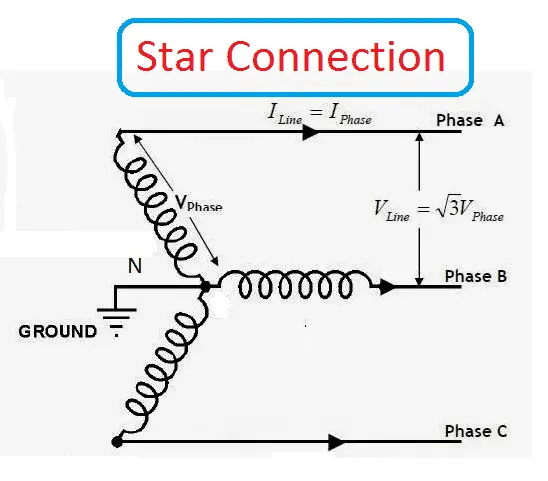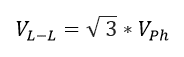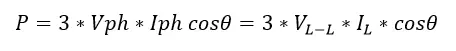# What is Star Connection in Three Phase Power System

## What is Star Connection:

Star connection or Y connection is an arrangement of three of same or different passive components (resistor, inductor, capacitor). This arrangement is generally used in the three-phase power system to deliver the balanced load from the Alternating current (AC) voltage source. When the current flow through all three phases is equal then it is called as balanced current. And when the current flow through the three-phase system is not equal, then it is called an unbalanced current. In this case, during balanced condition, there will be no current flowing through the neutral line and hence there is no use of the neutral terminal. But when there will be unbalanced current flowing in the three-phase circuit, neutral is having a vital role. It will take the unbalanced current through to the ground and protect the transformer this is the principle is used in high voltage power transmission system also, Unbalanced current may affect the transformer and it may also cause the physical damage to the transformer to avoid this star connection is preferred high voltage transformer for transferring power for long distance.Learn More:   Why India has 50 Hz Power System and US has 60 Hz 110 Volts Power System

Example: Electrical Motor, Electric alternator, three-phase heater, three-phase transformer.

### Star Connection Implementation:

Take three components i.e three Inductor, L1, L2 and L3. Connect each component’s one terminal to another as shown in the above figure.

Key points:

• Three phase balance star connection works under kickoff’s current rule. The algebraic sum of all current in a node is equal to zero.
• The line voltage is equal to 3 times of phase voltage• The line current is equal to phase current• Net power transfer in the three-phase system P isPower is an independent quantity of the circuit arrangement of the three-phase system. The net power in the circuit will be same in phase and line.

• Since there are three phases, so the multiple of 3 is made in the normal power equation and the PF is power factor. Power factor is a very important factor in three phase system and sometimes due to certain error, it is corrected by using capacitors.
Learn More:   What is Electric Field, Electric Field Intensity, Electric Field Density

• The insulation cost can be reduced by decreasing the phase voltage.
• Mainly, this arrangement is used High starting current/torque application such as grinder, chain feeder, Mixer.
• High voltage Power transmission
• Increases the system stability or protecting electrical equipment from heavy fault by turning the Fault current into the ground.
• The effective way of Power can be saved by reducing the voltage of the motor.